### SOLUTIONS TO U-SUBSTITUTION

SOLUTION 1 : Integrate. Let

u = x2+5x

so that

du = (2x+5) dx .

Substitute into the original problem, replacing all forms of x, getting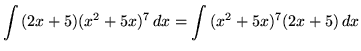.

SOLUTION 2 : Integrate. Let

u = 3-x

so that

du = (-1) dx ,

or

(-1) du = dx .

Substitute into the original problem, replacing all forms of x, getting.

SOLUTION 3 : Integrate. Let

u = 7x+9

so that

du = 7 dx ,

or

(1/7) du = dx .

Substitute into the original problem, replacing all forms of x, getting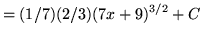.

SOLUTION 4 : Integrate. Let

u = 1+x4

so that

du = 4x3 dx ,

or

(1/4) du = x3 dx .

Substitute into the original problem, replacing all forms of x, getting(Do not make the following VERY COMMON MISTAKE :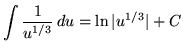. Why is this INCORRECT ?)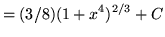.

SOLUTION 5 : Integrate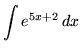. Let

u = 5x+2

so that

du = 5 dx ,

or

(1/5) du = dx .

Substitute into the original problem, replacing all forms of x, getting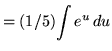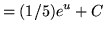.

SOLUTION 6 : Integrate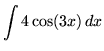. Let

u = 3x

so that

du = 3 dx ,

or

(1/3) du = dx .

Substitute into the original problem, replacing all forms of x, getting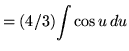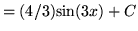.

SOLUTION 7 : Integrate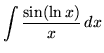. Letso that.

Substitute into the original problem, replacing all forms of x, getting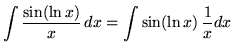.